# How to Calculate and Solve for Average Outflow Rate | Hydrology II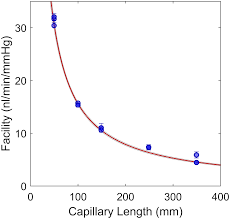The image above represents average outflow rate.

To compute for average outflow rate, three essential parameters are needed and these parameters are Average inflow rate (I), Change in storage during routing time (Δs) and Routing Time (Δt).

The formula for calculating average outflow rate:

O = I – Δs / Δt

Where:

O = Average Outflow Rate
I = Average Inflow Rate
Δs = Change in Storage during Routing Time
Δt = Routing Time

Let’s solve an example;
Given that the average inflow rate is 5, the change in storage during routing time is 2 and routing time is 10. Find the average outflow rate?

This implies that;

I = Average Inflow Rate = 5
Δs = Change in Storage during Routing Time = 2
Δt = Routing Time = 10

O = I – Δs / Δt
O = 5 – 2 / 10
O = 5 – 0.2
O = 4.8

Therefore, the average outflow rate is 4.8.

Calculating the Average Inflow Rate when the Average Outflow Rate, the Change in Storage during Routing Time and the Routing Time is Given.

I = O + Δs / Δt

Where;

I = Average Inflow Rate
O = Average Outflow Rate
Δs = Change in Storage during Routing Time
Δt = Routing Time

Let’s solve an example;
Find the Average Inflow Rate when the average outflow rate is 40, change in storage during routing time is 10 and the routing time is 5.

This implies that;

I = Average Inflow Rate = 40
Δs = Change in Storage during Routing Time = 10
Δt = Routing Time = 5

I = O + Δs / Δt
I = 40 + 105
I = 40 – 2
I = 38

Therefore, the average inflow rate is 38.

Calculating the Change in Storage during Routing Time when the Average Inflow Rate, the Average Outflow Rate and the Routing Time is Given.

Δs = (I – O) Δt

Where;

Δs = Change in Storage during Routing Time
I = Average Inflow Rate
O = Average Outflow Rate
Δt = Routing Time

Let’s solve an example;
Find the change in storage during routing time when the average inflow rate is 15, the average outflow rate is 10 and the routing time is 20.

This implies that;

I = Average Inflow Rate = 15
O = Average Outflow Rate = 10
Δt = Routing Time = 20

Δs = (I – O) Δt
Δs = (15 – 10) 20
Δs = (5) 20
Δs = 100

Therefore, the change in storage during routing time is 100.

Calculating for Routing Time when the Average Inflow Rate, the Average Outflow Rate and the Change in Storage During Routing Time is Given.

Δt = Δs / (I – O)

Where;

Δt = Routing Time
I = Average Inflow Rate
O = Average Outflow Rate
Δs = Change in Storage during Routing Time

Let’s solve an example;
Find the routing time when the average inflow rate is 25, the average outflow rate is 15 and the change in storage during routing time is 30.

This implies that;

I = Average Inflow Rate = 25
O = Average Outflow Rate = 15
Δs = Change in Storage during Routing Time = 30

Δt = Δs / (I – O)
Δt = 30 / (25 – 15)
Δt = 30 / (10)
Δt = 3

Therefore, the routing time is 3.

Nickzom Calculator – The Calculator Encyclopedia is capable of calculating the average outflow rate.

To get the answer and workings of the average outflow rate using the Nickzom Calculator – The Calculator Encyclopedia. First, you need to obtain the app.

You can get this app via any of these means:

To get access to the professional version via web, you need to register and subscribe for NGN 2,000 per annum to have utter access to all functionalities.
You can also try the demo version via https://www.nickzom.org/calculator

Apple (Paid) – https://itunes.apple.com/us/app/nickzom-calculator/id1331162702?mt=8
Once, you have obtained the calculator encyclopedia app, proceed to the Calculator Map, then click on Agricultural under Engineering.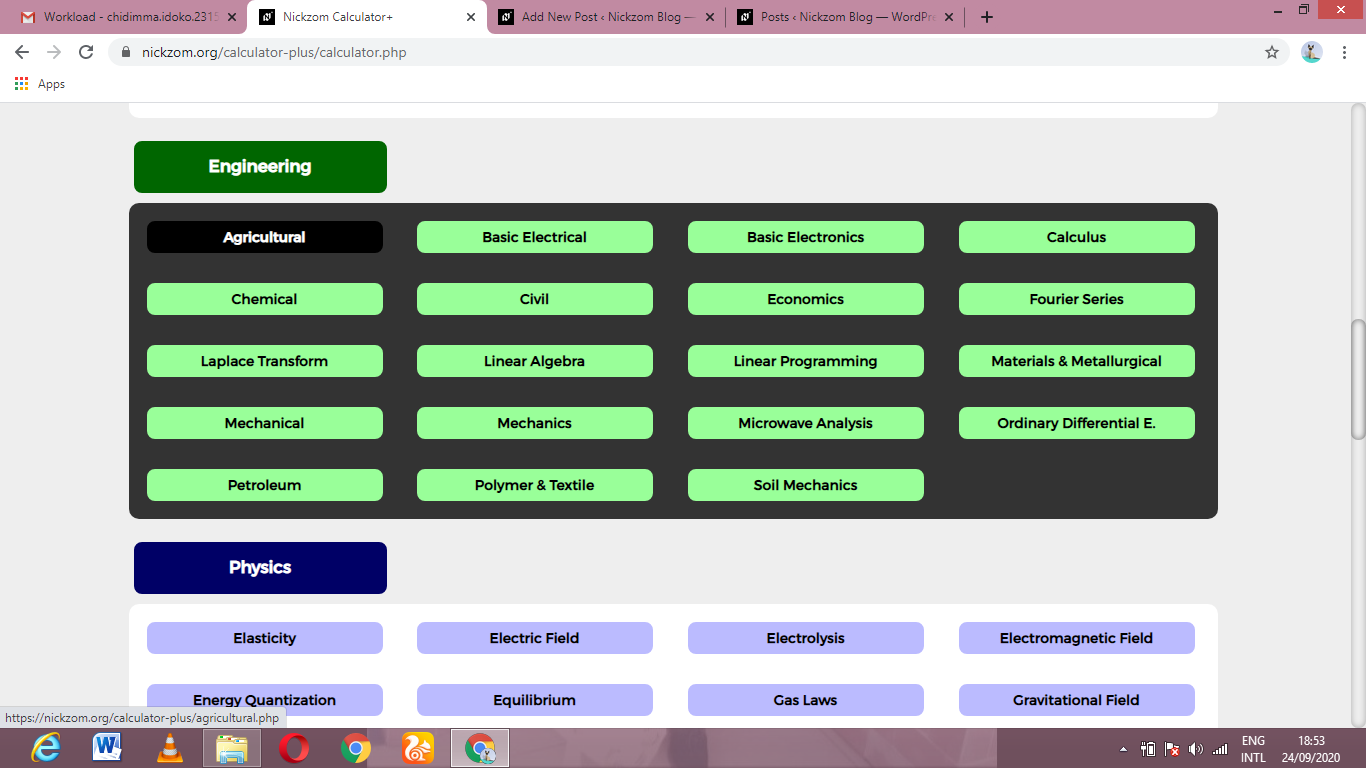Now, Click on Hydrology II under AgriculturalNow, Click on Average Outflow Rate under Hydrology II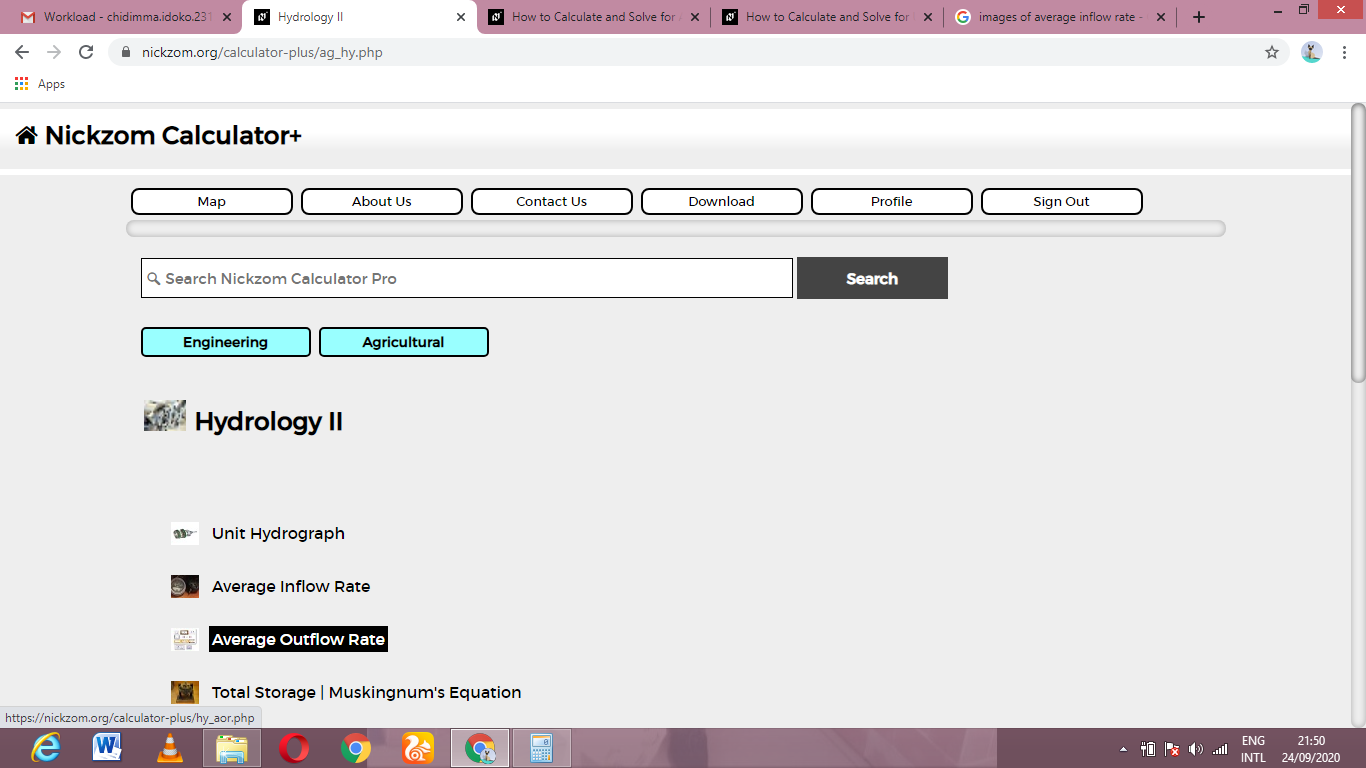The screenshot below displays the page or activity to enter your values, to get the answer for the average outflow rate according to the respective parameters which is the Average inflow rate (I), Change in storage during routing time (Δs) and Routing Time (Δt).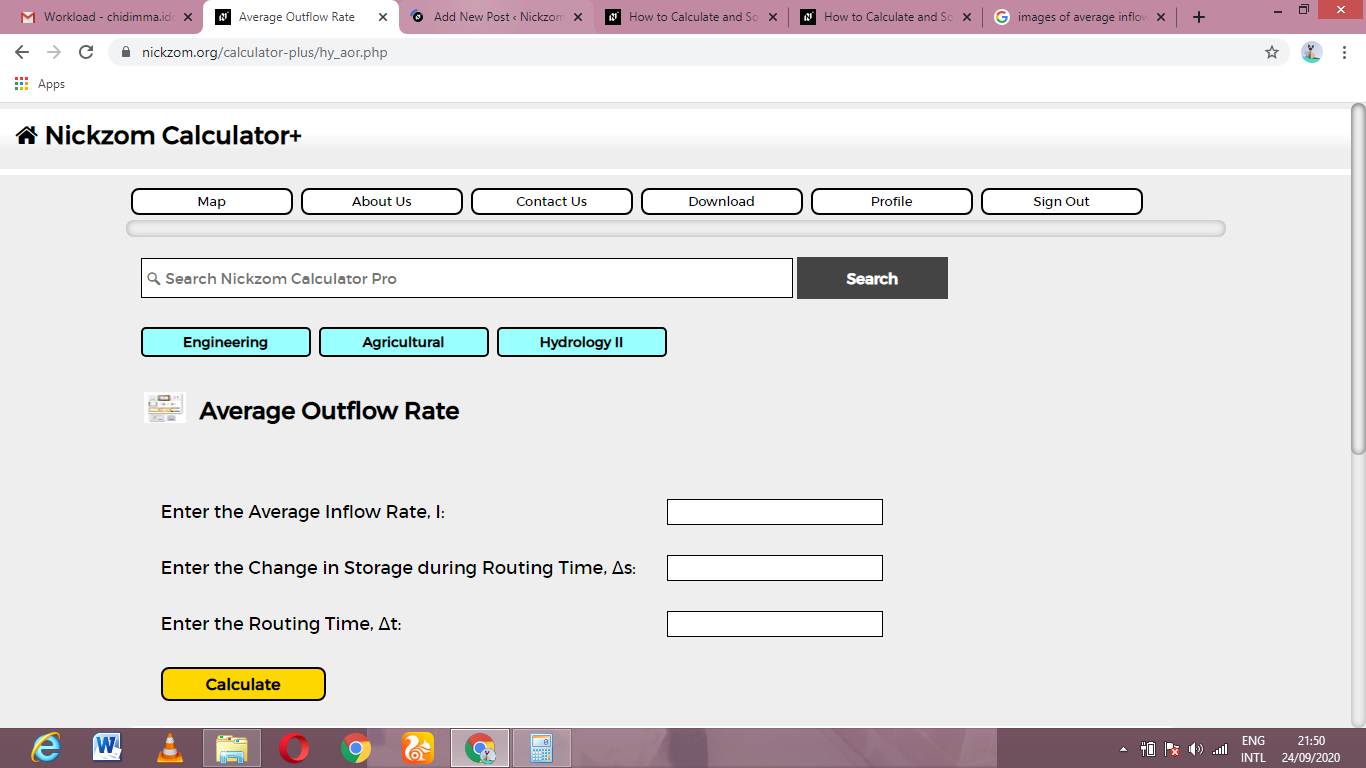Now, enter the values appropriately and accordingly for the parameters as required by the Average inflow rate (I) is 5, Change in storage during routing time (Δs) is 2 and Routing Time (Δt) is 10.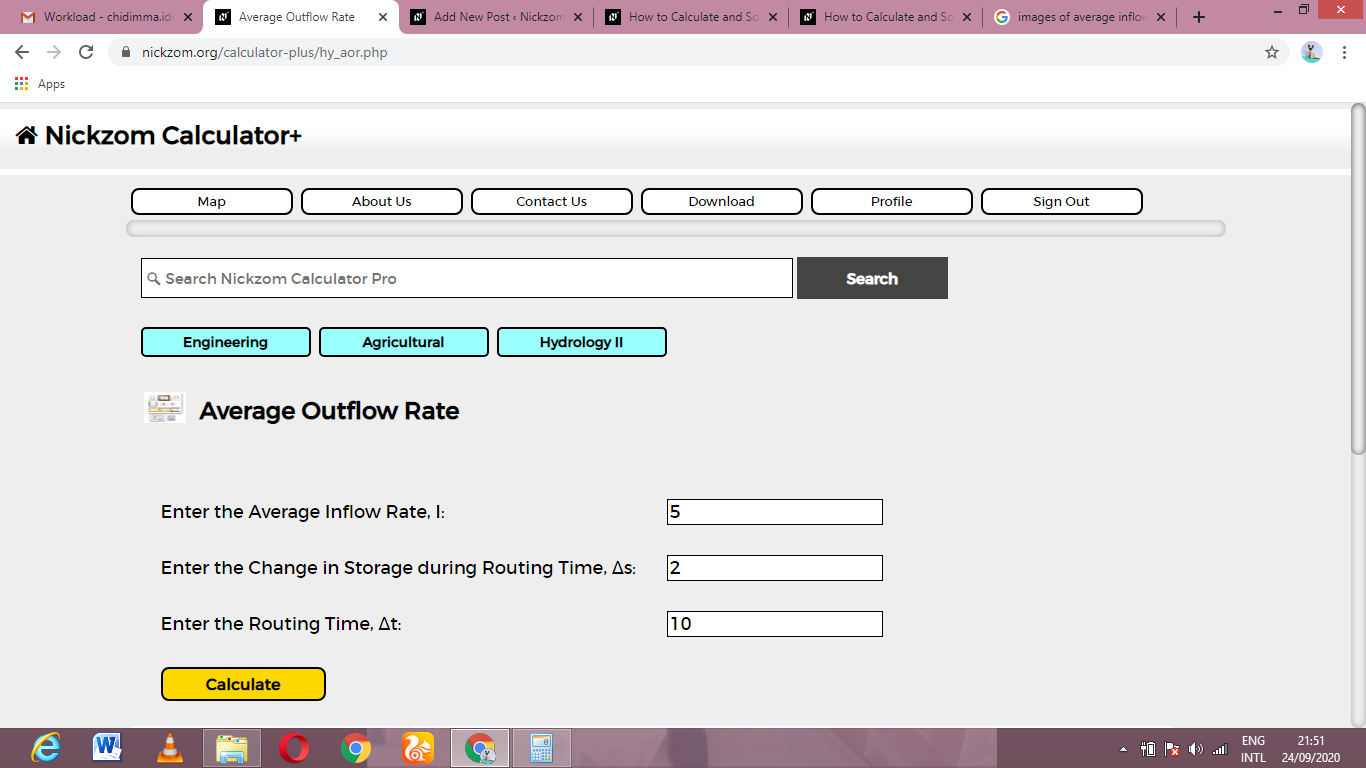Finally, Click on Calculate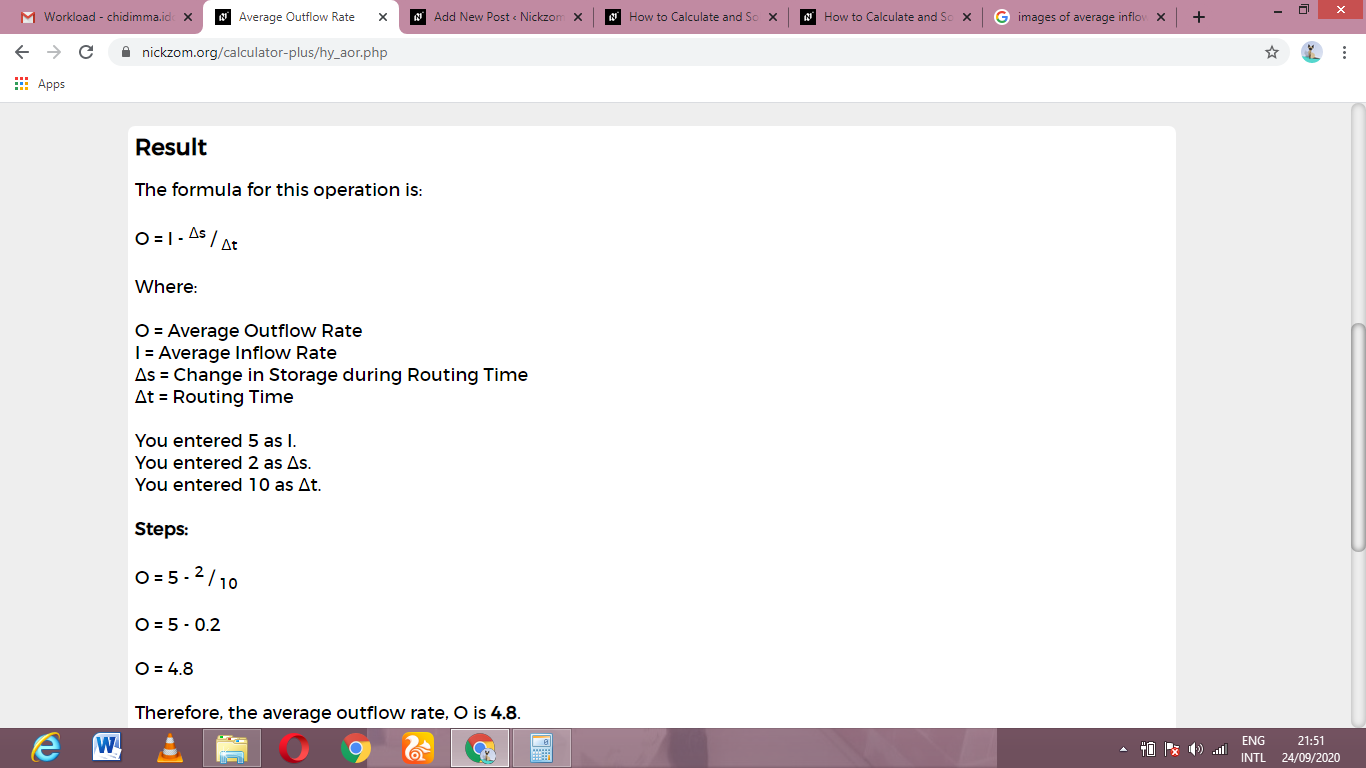As you can see from the screenshot above, Nickzom Calculator– The Calculator Encyclopedia solves for the average outflow rate and presents the formula, workings and steps too.